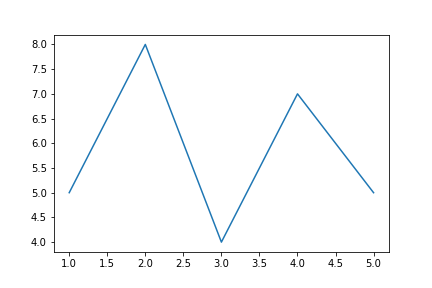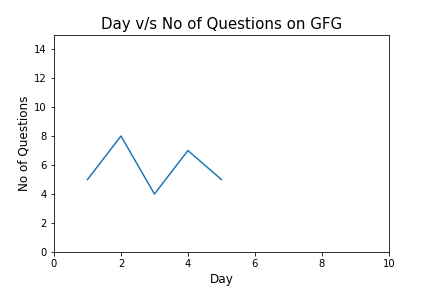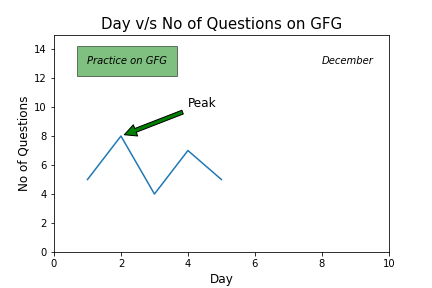Skip to content
Related Articles
How to add text to Matplotlib?
• Last Updated : 03 Jan, 2021

Matplotlib is a plotting library in Python to visualize data, inspired by MATLAB, meaning that the terms used (Axis, Figure, Plots) will be similar to those used in MATLAB. Pyplot is a module within the Matplotlib library which is a shell-like interface to Matplotlib module.

It provides almost any kind of plot that we can think of. In this post, we will focus on a more specific topic which is adding text on matplotlib plot. The following commands are used to create text in the matplotlib plot.

We will see each of the commands one by one, first, let’s create a basic plot of Day v/s Question on which we will add various text objects.

Code:

## Python3

 `# Code to add text on matplotlib`` ` `# Importing library``import` `matplotlib.pyplot as plt`` ` `# Creating x-value and y-value of data``x ``=` `[``1``, ``2``, ``3``, ``4``, ``5``]``y ``=` `[``5``, ``8``, ``4``, ``7``, ``5``]`` ` `# Creating figure``fig ``=` `plt.figure()`` ` `# Adding axes on the figure``ax ``=` `fig.add_subplot(``111``)`` ` `# Plotting data on the axes``ax.plot(x, y)`` ` `plt.show()`

Output:The output plot looks very simple. Now, let’s see some text commands to add it on our plot.

• set_title() is used to add the title of axes. The first and mandatory argument is the title you want to give and the rest are optional to formate it.
• Similarly, set_xlabel() and set_ylabel() are used to add titles to x-axis and y-axis. It also takes title as an argument.

It is better to adjust the range on y-axis so that we can have some space to add text later on. For this, we will use ax.axis() which allows specifying value ranges (the first two for x-axis and the other two for y-axis).

Now, let’s add its title and names of x-axis and y-axis.

Code:

## Python3

 `# Adding title``ax.set_title(``'Day v/s No of Questions on GFG'``, fontsize``=``15``)`` ` `# Adding axis title``ax.set_xlabel(``'Day'``, fontsize``=``12``)``ax.set_ylabel(``'No of Questions'``, fontsize``=``12``)`` ` `# Seting axis limits``ax.axis([``0``, ``10``, ``0``, ``15``])`

Output:Now, it looks better than the previous version. It’s the time to add text to our plot. First, let’s see about them.

axes.text() is used to add text at an arbitrary location of the Axes. For this we need to specify the location of the text and of course what the text is. For instance, the following code will add “Practice on GFG” text. It will located according to the point whose coordinates are specified ([1,13] in this case). The parameter bbox is used to capture the text with a box. As argument to bbox parameter, we pass a dictionary which includes formatting styles.

Code:

## Python3

 `# Adding text on the plot.``ax.text(``1``, ``13``, ``'Practice on GFG'``, style``=``'italic'``, bbox``=``{``        ``'facecolor'``: ``'grey'``, ``'alpha'``: ``0.5``, ``'pad'``: ``10``})`

If we want to do not have to box the text then simply do not assign anything to the bbox parameter. The following code adds the specified text without a box.

Code:

## Python3

 `# Adding text without box on the plot.``ax.text(``8``, ``13``, ``'December'``, style``=``'italic'``)`

We can also add text with annotations.

axes.annotate() is used to add an annotation, with an optional arrow, at an arbitrary location of the Axes. Its xy parameter contains the coordinates for arrow and xytext parameter specifies the location of the text. Arrowprops parameter is used to style the arrow.

For instance, we can mark the peak value of the Day-Question data with an annotation.

Code:

## Python3

 `# Adding annotation on the plot.``ax.annotate(``'Peak'``, xy``=``(``2``, ``8``), xytext``=``(``4``, ``10``), fontsize``=``12``,``            ``arrowprops``=``dict``(facecolor``=``'green'``, shrink``=``0.05``))`

Let’s put all this together and see the final code.

Code:

## Python3

 `# Code to add text on matplotlib`` ` `# Importing library``import` `matplotlib.pyplot as plt`` ` `# Creating x-value and y-value of data``x ``=` `[``1``, ``2``, ``3``, ``4``, ``5``]``y ``=` `[``5``, ``8``, ``4``, ``7``, ``5``]`` ` `# Creating figure``fig ``=` `plt.figure()`` ` `# Adding axes on the figure``ax ``=` `fig.add_subplot(``111``)`` ` `# Plotting data on the axes``ax.plot(x, y)`` ` `# Adding title``ax.set_title(``'Day v/s No of Questions on GFG'``, fontsize``=``15``)`` ` `# Adding axis title``ax.set_xlabel(``'Day'``, fontsize``=``12``)``ax.set_ylabel(``'No of Questions'``, fontsize``=``12``)`` ` `# Seting axis limits``ax.axis([``0``, ``10``, ``0``, ``15``])`` ` `# Adding text on the plot.``ax.text(``1``, ``13``, ``'Practice on GFG'``, style``=``'italic'``, bbox``=``{``        ``'facecolor'``: ``'green'``, ``'alpha'``: ``0.5``, ``'pad'``: ``10``})`` ` `# Adding text without box on the plot.``ax.text(``8``, ``13``, ``'December'``, style``=``'italic'``)`` ` `# Adding annotation on the plot.``ax.annotate(``'Peak'``, xy``=``(``2``, ``8``), xytext``=``(``4``, ``10``), fontsize``=``12``,``            ``arrowprops``=``dict``(facecolor``=``'green'``, shrink``=``0.05``))`` ` `plt.show()`

Output:Attention geek! Strengthen your foundations with the Python Programming Foundation Course and learn the basics.

To begin with, your interview preparations Enhance your Data Structures concepts with the Python DS Course. And to begin with your Machine Learning Journey, join the Machine Learning – Basic Level Course

My Personal Notes arrow_drop_up